# Karnataka Board SSLC Class 10 Science Question Paper with Solutions 2016

Students should start their exam preparation by solving Karnataka Board SSLC Class 10 Science previous year question papers of 2016. By doing so, they can understand the exact question paper pattern, important topics, weightage of each question, etc. Knowing the question paper pattern will help them students to prepare themselves accordingly. To help students in this article, we have provided solutions of KSEEB Class 10 Science question papers of 2016. All the answers are solved correctly in detail using simple language.

By solving the previous year question papers students can also work on their time management skills. It will help them to complete the final question on time. Students should refer to the solutions provided below, once they complete solving the complete question paper. This will help them to clear their doubts.

### KSEEB Class 10 Science Question Paper With Solution 2016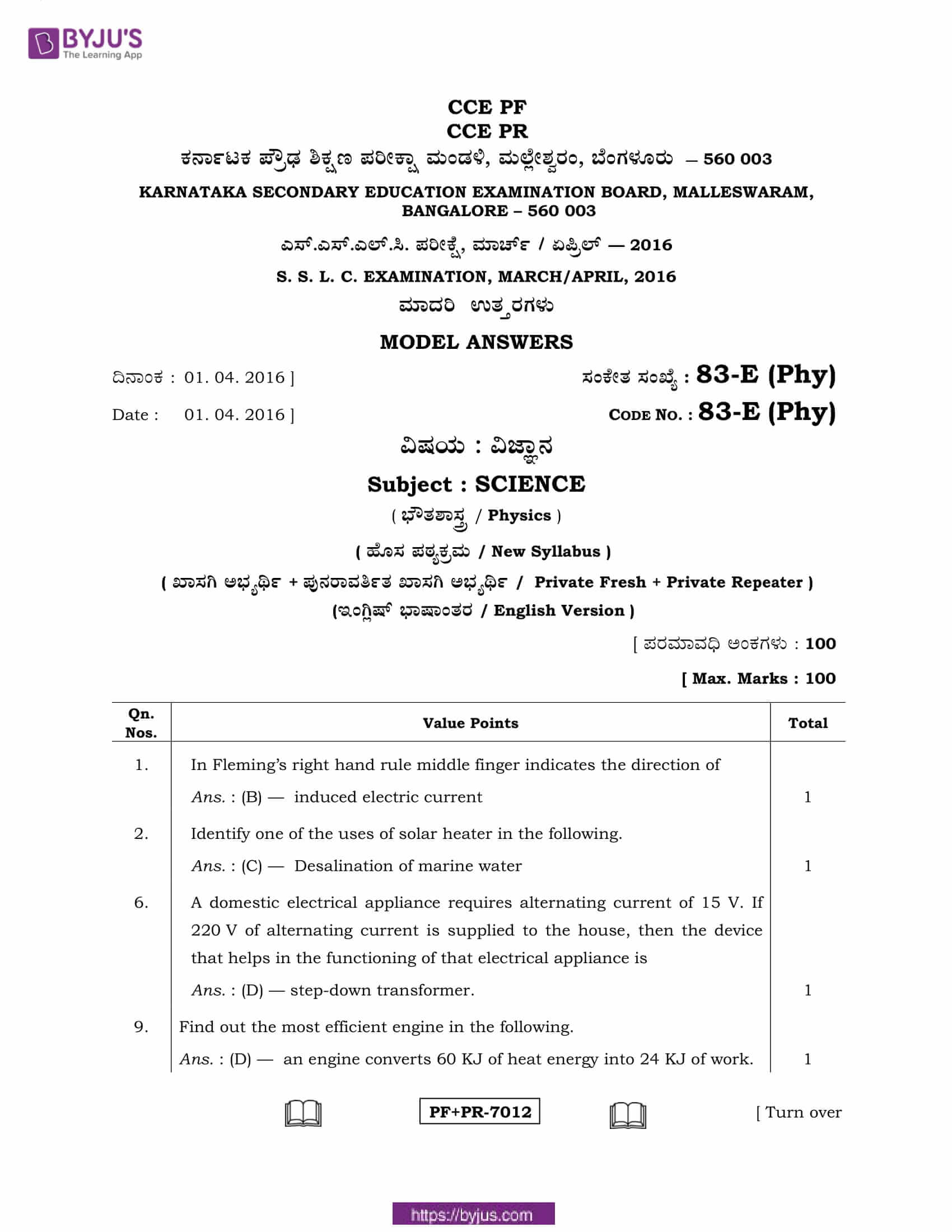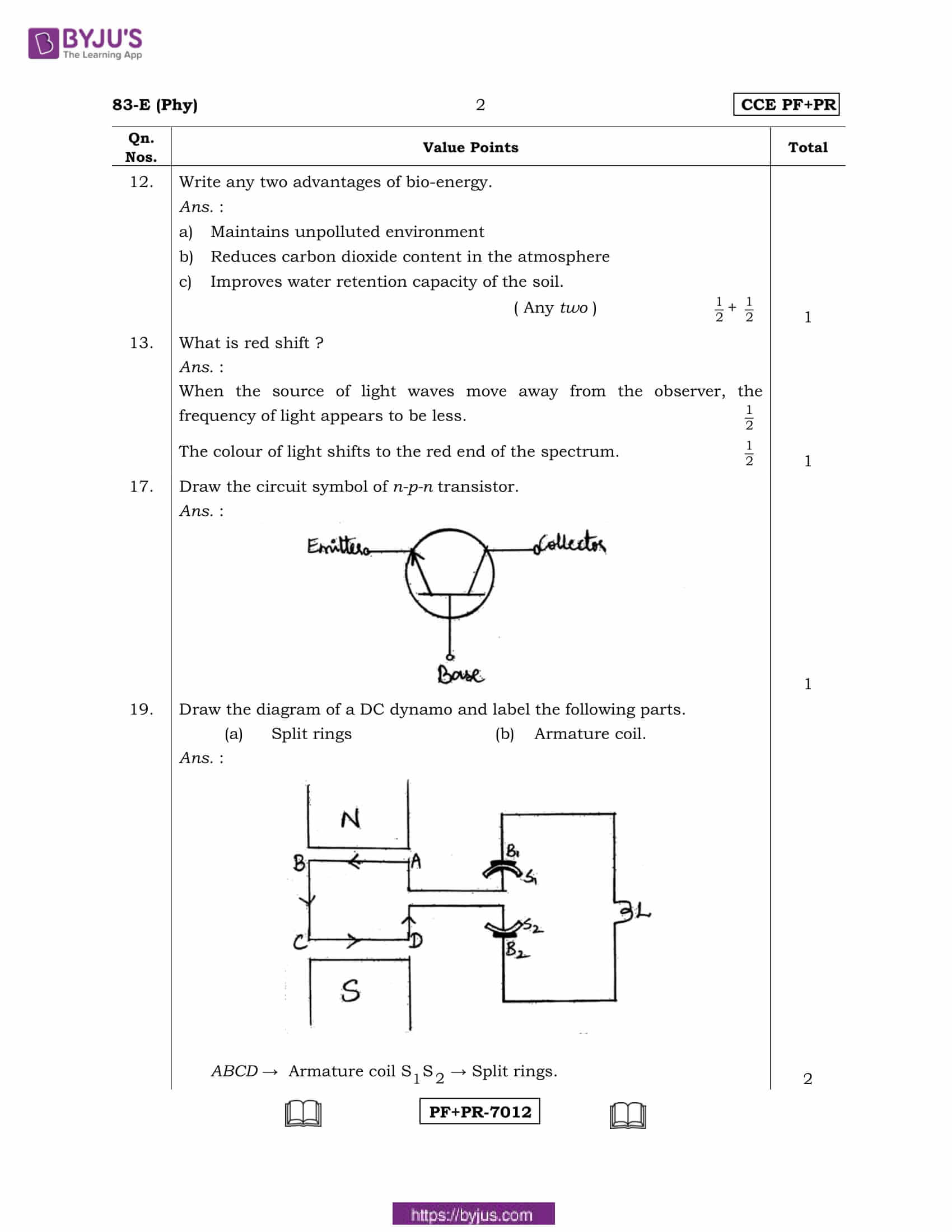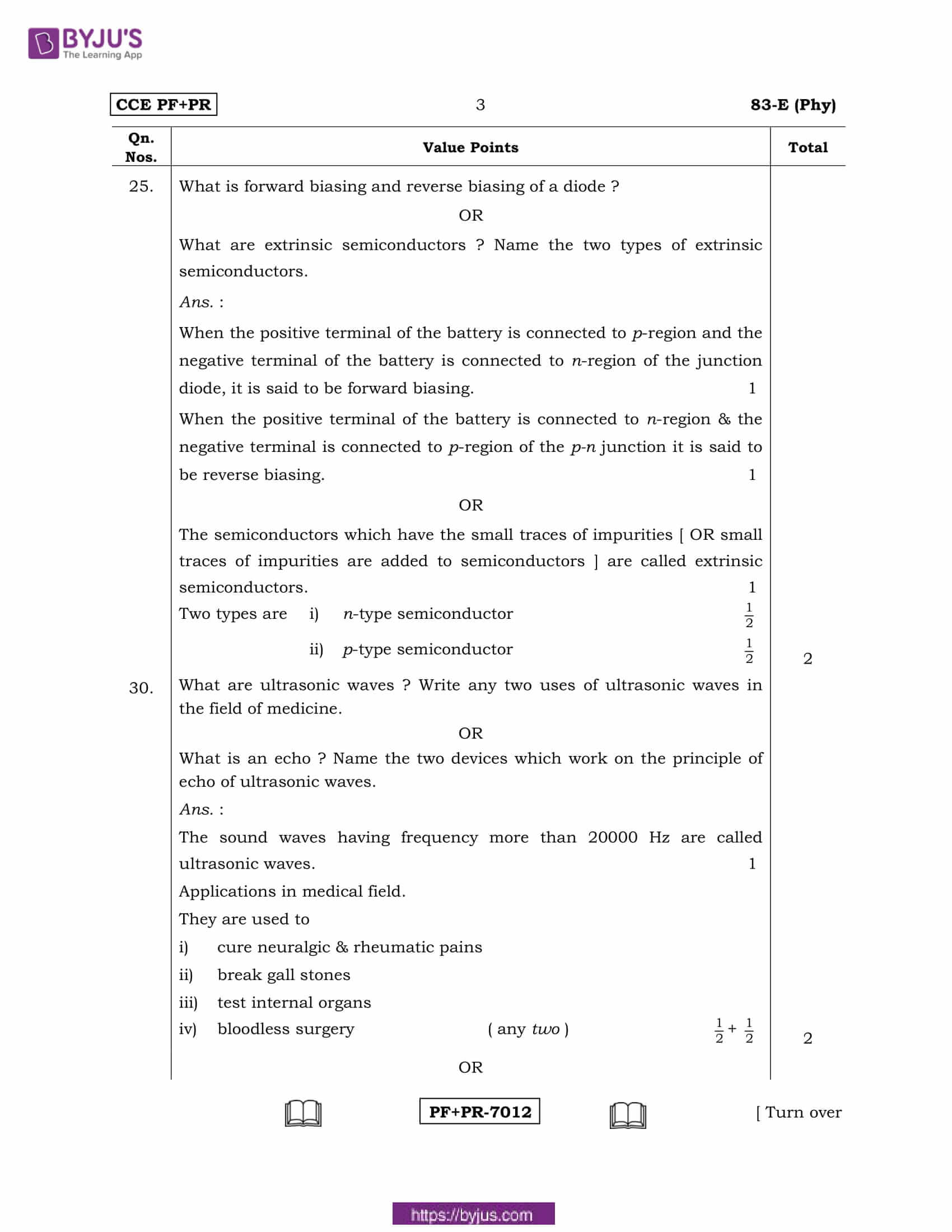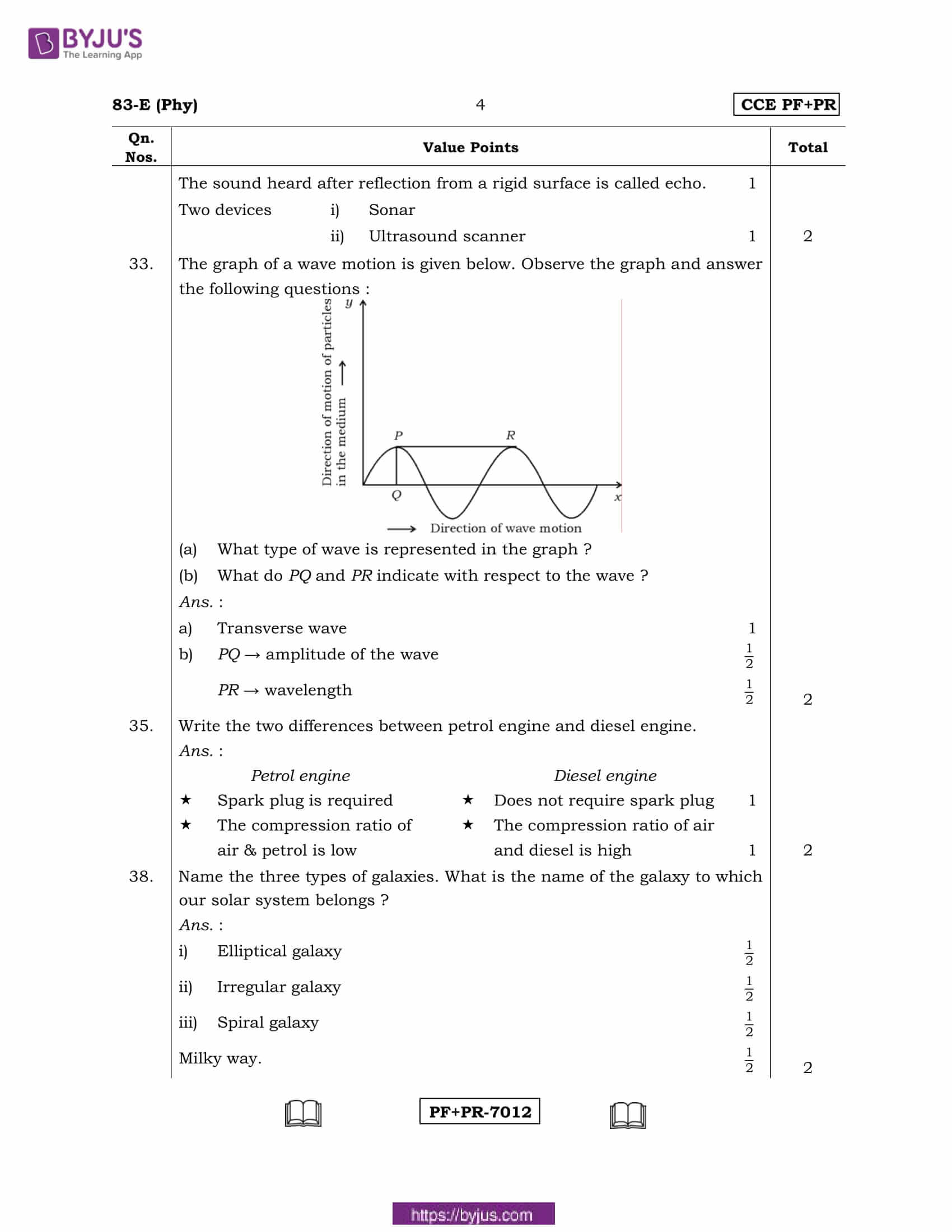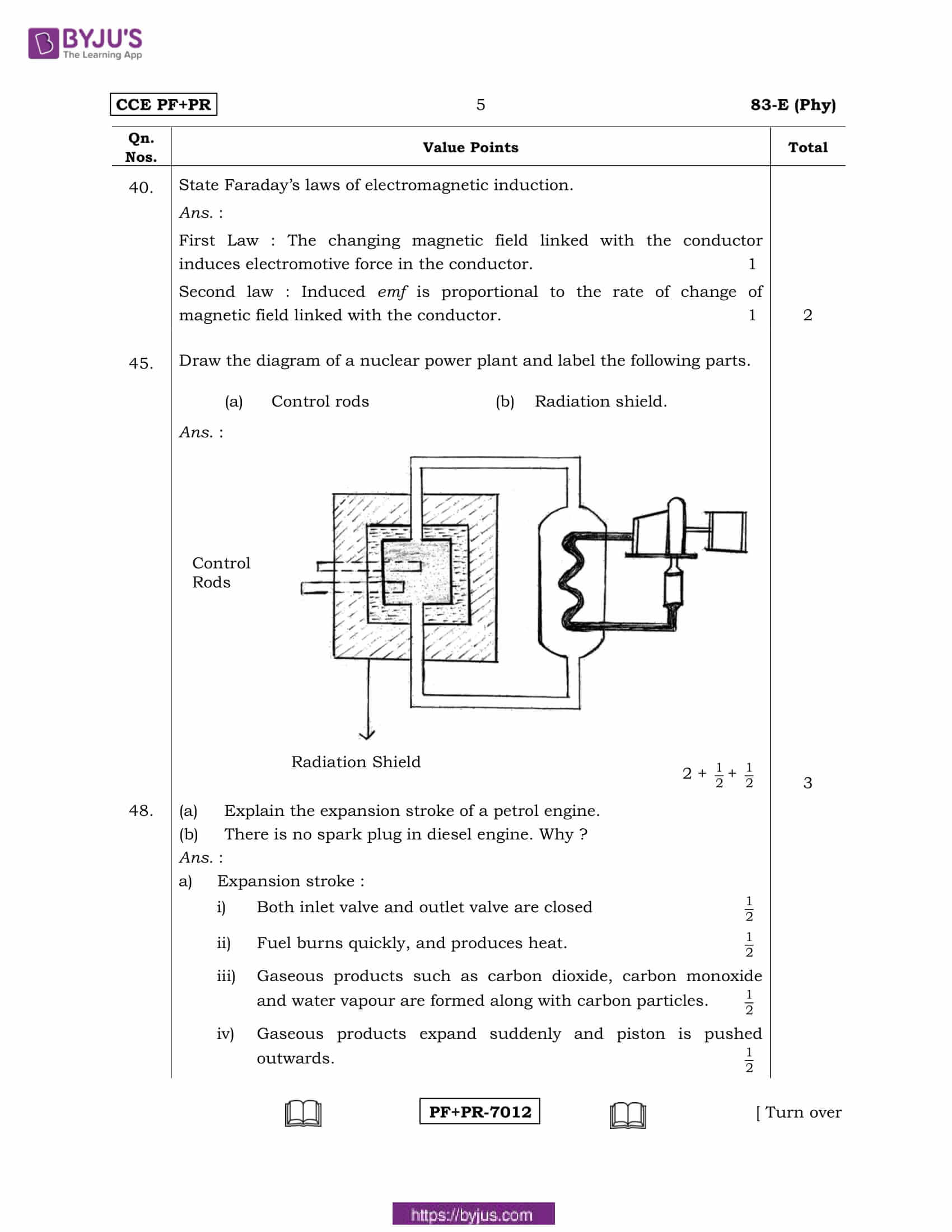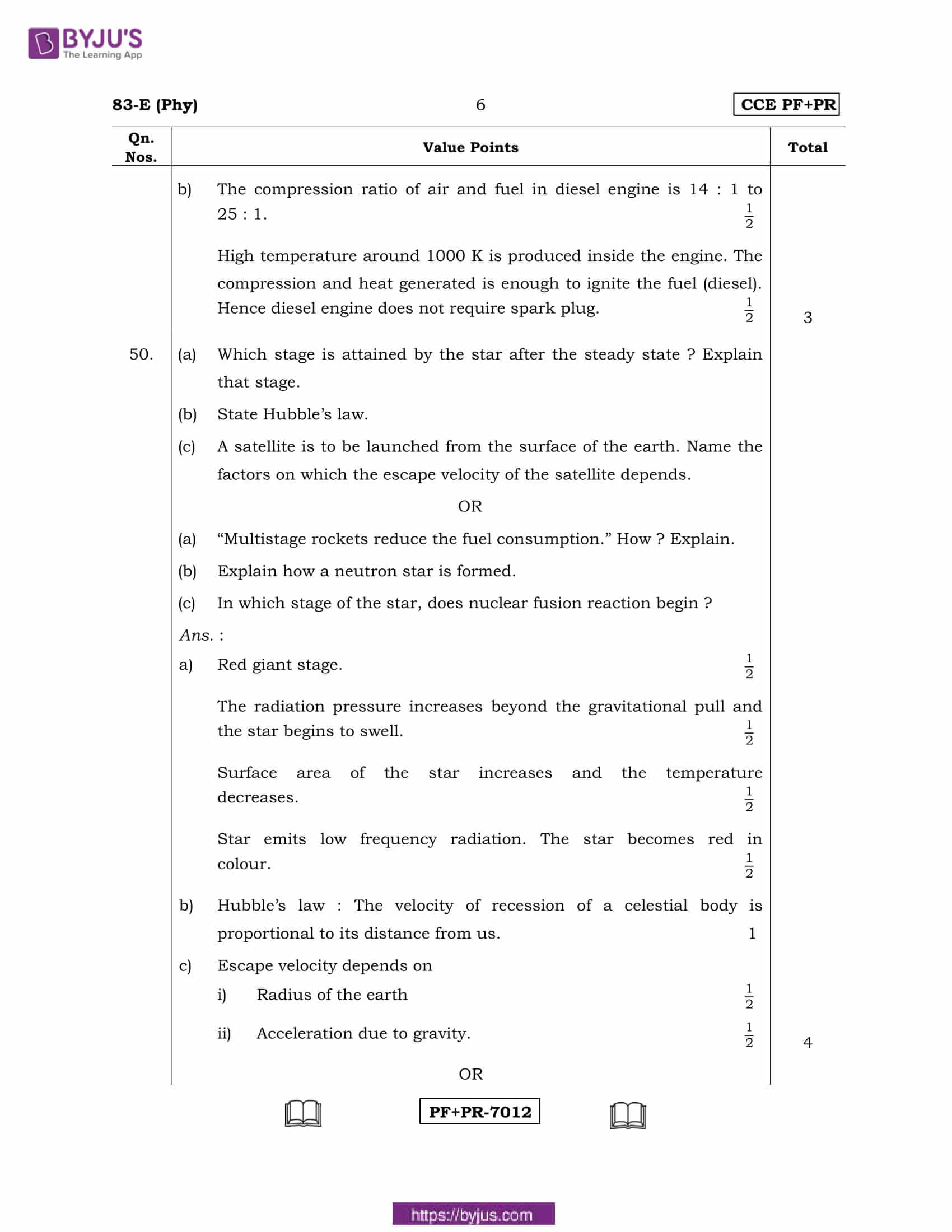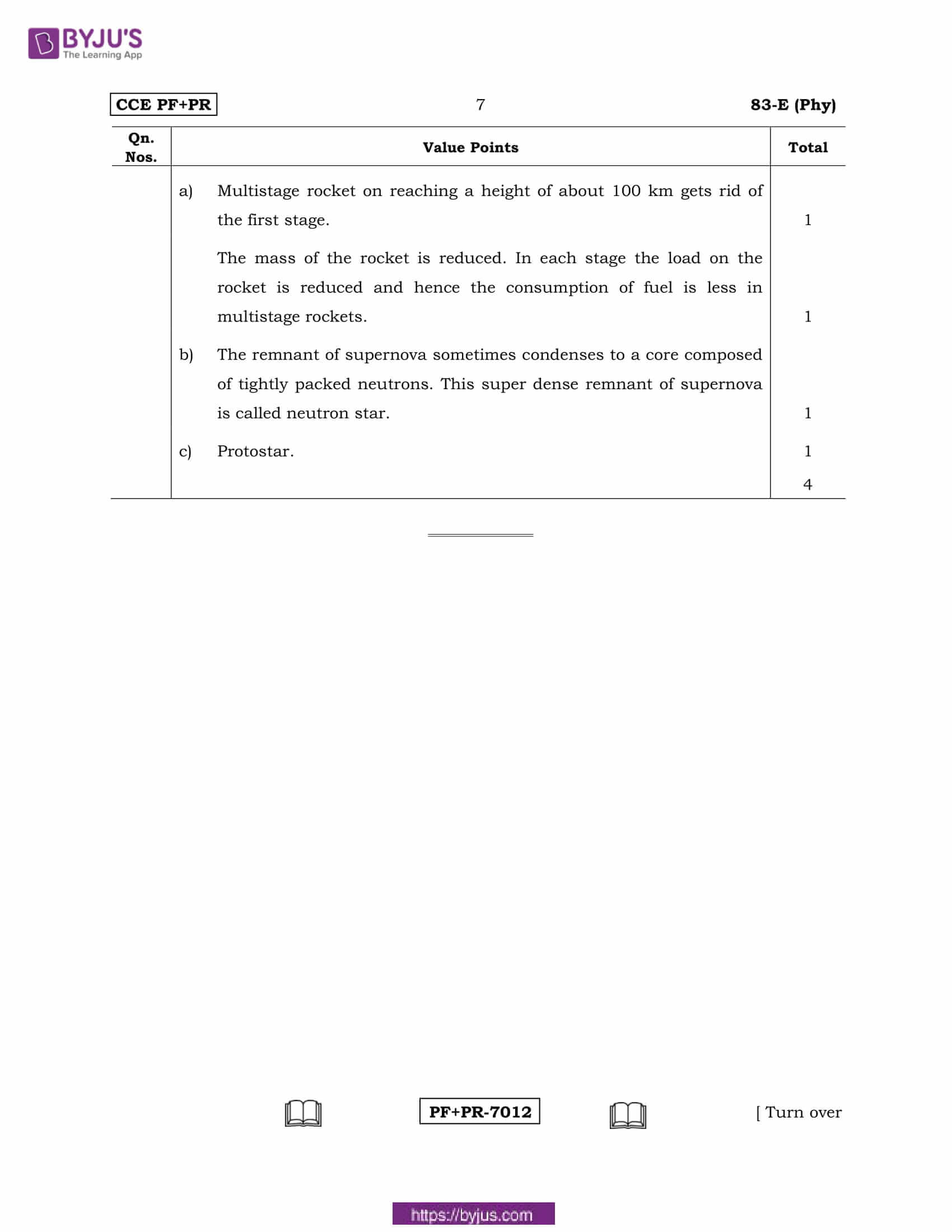Four alternatives are given for each of the following questions / incomplete statements. Only one of them is correct or most appropriate. Choose the correct alternative and write the complete answer along with its letter in the space provided against each question.

1. In Fleming’s right hand rule middle finger indicates the direction of

(A) magnetic field

(B) induced electric current

(C) mechanical energy

(D) motion of the conductor.

Answer: (B) — induced electric current

2. Identify one of the uses of solar heater in the following.

(A) Conversion of solar energy into electrical energy

(B) Providing energy for artificial satellites

(C) Desalination of marine water

(D) Using in automatic street lights.

Answer: (C) — Desalination of marine water 1

6. A domestic electrical appliance requires alternating current of 15 V. If 220 V of alternating current is supplied to the house, then the device that helps in the functioning of that electrical appliance is

(A) induction coil

(B) step-up transformer

(C) AC dynamo

(D) step-down transformer.

9. Find out the most efficient engine in the following.

(A) an engine converts 80 KJ of heat energy into 20 KJ of work

(B) an engine converts 50 KJ of heat energy into 15 KJ of work

(C) an engine converts 30 KJ of heat energy into 6 KJ of work

(D) an engine converts 60 KJ of heat energy into 24 KJ of work.

Answer: (D) — an engine converts 60 KJ of heat energy into 24 KJ of work.

12. Write any two advantages of bio-energy.

a) Maintains unpolluted environment

b) Reduces carbon dioxide content in the atmosphere

c) Improves water retention capacity of the soil.

13. What is red shift?

Answer: When the source of light waves move away from the observer, the frequency of light appears to be less. The colour of light shifts to the red end of the spectrum.

17. Draw the circuit symbol of n-p-n transistor.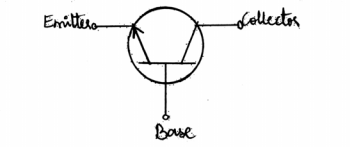19. Draw the diagram of a DC dynamo and label the following parts.

(a) Split rings

(b) Armature coil.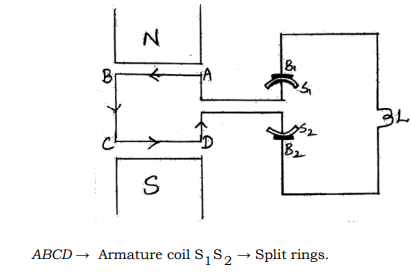25. What is forward biasing and reverse biasing of a diode?

OR

What are extrinsic semiconductors ? Name the two types of extrinsic semiconductors.

Answer: When the positive terminal of the battery is connected to p-region and the negative terminal of the battery is connected to n-region of the junction diode, it is said to be forward biasing.

When the positive terminal of the battery is connected to n-region & the negative terminal is connected to p-region of the p-n junction it is said to be reverse biasing.

Answer: The semiconductors which have the small traces of impurities [OR small traces of impurities are added to semiconductors] are called extrinsic semiconductors. Two types are

i) n-type semiconductor

ii) p-type semiconductor

30. What are ultrasonic waves ? Write any two uses of ultrasonic waves in the field of medicine.

OR

What is an echo ? Name the two devices which work on the principle of echo of ultrasonic waves.

Answer: The sound waves having frequency more than 20000 Hz are called ultrasonic waves.

Applications in medical field. They are used to

i) cure neuralgic & rheumatic pains

ii) break gall stones

iii) test internal organs

iv) bloodless surgery

Answer: The sound heard after reflection from a rigid surface is called echo. Two devices

i) Sonar

ii) Ultrasound scanner

33. The graph of a wave motion is given below. Observe the graph and answer the following questions: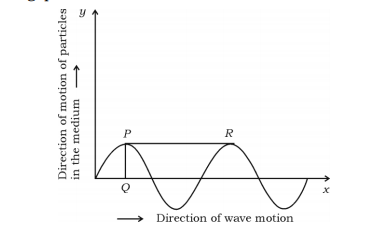(a) What type of wave is represented in the graph ?

(b) What do PQ and PR indicate with respect to the wave ?

Answer b: PQ → amplitude of the wave

PR → wavelength

35. Write the two differences between petrol engine and diesel engine.

Answer: The two differences between petrol engine and diesel engine are:

 Petrol Engine Diesel Engine Spark plug is required Does not require spark plug The compression ratio of air & petrol is low The compression ratio of air and diesel is high

38. Name the three types of galaxies. What is the name of the galaxy to which our solar system belongs?

Answer: The three types of galaxies are:

i) Elliptical galaxy

ii) Irregular galaxy

iii) Spiral galaxy 2

Milky Way is the name of the galaxy to which our solar system belongs to.

40. State Faraday’s laws of electromagnetic induction.

• First Law : The changing magnetic field linked with the conductor induces electromotive force in the conductor.
• Second law : Induced emf is proportional to the rate of change of magnetic field linked with the conductor.

45. Draw the diagram of a nuclear power plant and label the following parts.

(a) Control rods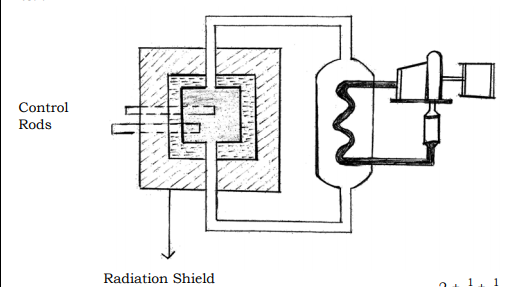38. (a) Explain the expansion stroke of a petrol engine.

(b) There is no spark plug in diesel engine. Why ?

i) Both inlet valve and outlet valve are closed

ii) Fuel burns quickly, and produces heat.

iii) Gaseous products such as carbon dioxide, carbon monoxide and water vapour are formed along with carbon particles.

iv) Gaseous products expand suddenly and piston is pushed outwards.

Answer b: There is no spark plug in diesel engine

• The compression ratio of air and fuel in diesel engine is 14:1 to 25:1.
• High temperature around 1000 K is produced inside the engine. The compression and heat generated is enough to ignite the fuel (diesel). Hence, diesel engine does not require spark plug.

50. (a) Which stage is attained by the star after the steady state ? Explain that stage.

(b) State Hubble’s law.

(c) A satellite is to be launched from the surface of the earth. Name the factors on which the escape velocity of the satellite depends.

OR

(a) “Multistage rockets reduce the fuel consumption.” How? Explain.

(b) Explain how a neutron star is formed.

(c) In which stage of the star, does nuclear fusion reaction begin?

• The radiation pressure increases beyond the gravitational pull and the star begins to swell.
• Surface area of the star increases and the temperature decreases.
• Star emits low frequency radiation. The star becomes red in colour.

Answer b: Hubble’s law: The velocity of recession of a celestial body is proportional to its distance from us.

Answer c: Escape velocity depends on